### Displacement and Strain: Material Time Derivative

### Material Time Derivative

For time dependent deformations, the material time derivative, is the derivative of scalar, vector or tensor fields as a function of the material point with respect to time. Let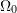and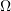represent the reference and the deformed configurations of a body embedded in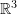. Let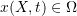represent the image of the vector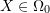at a certain point in time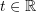. Letand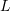and be the velocity and the velocity gradient fields. Let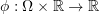,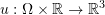and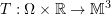be a scalar, a vector and a tensor field respectively. I.e.,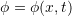,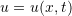and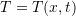. Then, the material time derivative of these fields, denotedwithbeing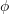,or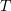is the total derivative of these quantities as follows: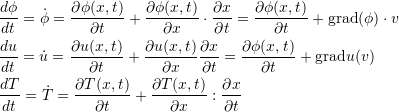Notice that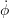is a scalar valued field,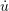is a vector valued field, while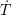is a tensor valued field. The components of these quantities are given by: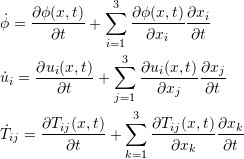In case where the fields are functions of the reference configuration, i.e.,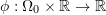,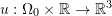and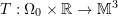are the respective scalar, vector and tensor fields, then, the material time derivative of these fields, denoted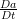withbeing,oris the total derivative of these quantities. However, since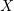is fixed, the only variable that appears in the derivation is time: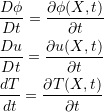And the components of these quantities are given by: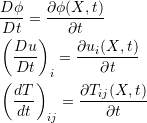#### Example:

In the following example, the scalar field temperature is used to illustrate the above concepts. Assuming a plate of width 4 units and height of 2 units is rotating around the origin with a speed of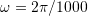rad/second. If the spatial distribution of the temperature in the medium is given by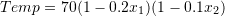units of temperature. If we assume that the speed of the plate is slow enough such that the plate assumes the temperature of the medium, then the material time derivative of the temperature is non zero.
The position function at timeis equal to: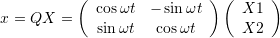whereThe spatial velocity field is given by the following vector function: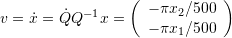The spatial gradient of temperature field is given by the following vector function: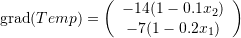The material time derivative of the temperature is given by the following scalar function: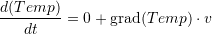The following tool draws the velocity vector field, the spatial gradient of temperature vector field and the contour plot of the material time derivative of the temperature. Change the value ofbetween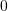and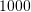units to see the effect on the position of the plate and the calculated fields.